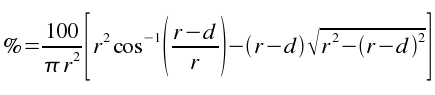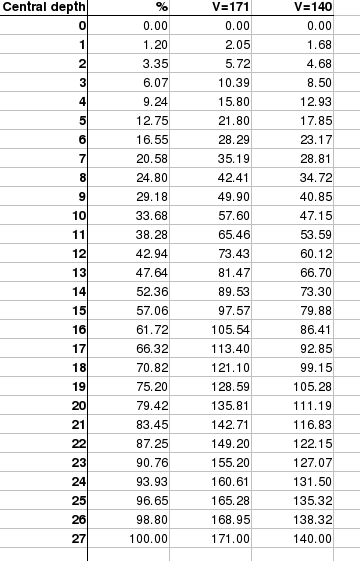SEARCH HOMEMath Central Quandaries & QueriesQuestion from Steve: I am not a math expert and want help seeing how much fuel is in a tank at one given time the tank that i have is 27 inches round. It is 69 inches long. laying length ways. it holds 140 gallons of fuel at fill up. I do know that it would hold less at the bottom of the tank an more in the center. Is there anybody that could just give me the figures by the inch without a formula. thanks for your timeHi Steve.

I did a similar calculation a couple of years ago. You can see the mathematical derivation here.

You appear to have a radius of 13.5 inches and a length of 69 inches. Since you are writing from the United States, I presume you know that 231 cubic inches equals a US gallon. So the volume of your tank is πr2L cubic inches. Thus according to the dimensions you provided, that is 3.14159(13.5)2(69) = 39506 cubic inches. That's 39506/231 = 171 gallons, not the 140 gallons you indicated. I think this discrepancy may be due to external dimensions compared to internal dimensions, or perhaps imprecise measurements.

The computations I did for Andy can be represented as a "percentage full" based on the inch level. I derived the following for your situation (using r as the radius and d as the central depth of liquid):I punched this into a spreadsheet using r = 13.5 and d going from 0 to 27. Then I used the two volumes (my calculation of 171 based on your measurements; and your given volume of 140 gallons). So here is a depth to volume table:Hope this helps,
Stephen La RocqueMath Central is supported by the University of Regina and The Pacific Institute for the Mathematical Sciences.